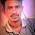In our previous topics, we have seen some important concepts such as Assumptions made in the Euler’s column theoryDifference between column and strutDifference between long columns and short columns and Failure of a column with the help of our previous posts.

Today we will see here one very important topic in strength of material i.e. Expression for crippling load when both the ends of the column are hinged with the help of this post.

Before going ahead, we must have to understand here the significance of crippling load or buckling load.

When a column will be subjected to axial compressive loads, there will be developed bending moment and hence bending stress in the column. Column will be bent due to this bending stress developed in the column.

Load at which column just bends or buckles will be termed as buckling or crippling load.
Let us consider a column AB of length L as displayed in following figure. Let us consider that both the ends of the column are hinged i.e. end A and end B are hinged.

Let us think that P is the load at which column just bends or buckles or we can also say that crippling load is P and we have displayed in following figure. Curve ACB indicates the condition of column after application of crippling load or when column buckles.
Now, we will consider one section at a distance x from end A and let us consider that y is the lateral deflection of the column as displayed in above figure.

Now we will determine the bending moment developed across the section and we can write it as mentioned here

Bending Moment, M = - P x y

We have taken negative sign here for bending moment developed due to crippling load across the section and we can refer the post for securing the information about the sign conventions used for bending moment for columns.

As we know the expression for bending moment from deflection equation and we can write as mentioned her.

Bending Moment, M = E.I [d2y/dx2]

We can also write here the equation after equating both expressions for bending moment mentioned above and we will have following equation
Above equation will also be termed as lateral deflection equation for column.
C1 and C2 are the constant of integration, now next step is to determine the value of constant of integration i.e. C1 and C2.

We will refer here one of our previous post i.e. End conditions for long columns and we will secure the value of constant of integration i.e. C1 and C2 by using the respective end conditions.

As we know that for long column with both the ends hinged, we will have following end conditions as mentioned here.

At x = 0, deflection y = 0
At x = L, deflection y = 0

Let us use the first end condition i.e. at x = 0, deflection y = 0 in above lateral deflection equation for column and we will have value of constant of integration i.e.C1.

At x = 0, deflection y = 0 and hence after using these value in lateral deflection equation for column, we will have constant of integration i.e.C1 = 0

Similarly, we will use second end condition i.e. at x = L, deflection y = 0 and constant of integration i.e.C1 = 0 in above lateral deflection equation for column and we will have value of constant of integration i.e.C2.

At x = L, deflection y = 0 and we have already determined constant of integration i.e.C1 = 0. Therefore, constant of integration i.e.C2 will be determined as displayed here in following figure.
Let us assume that C2 is zero, we have already concluded that C1 = 0 and in this situation lateral deflection of column i.e. y will also be zero.

We can also say from here that column will not be bend after application of crippling load P and as we know that this statement will never be true and hence our assumption of taking C2 = 0 is wrong.
So only one condition is left as displayed here

From here we will have expression for crippling load when both the ends of the column are hinged and we have displayed it in following figure.
Do you have suggestions? Please write in comment box.

We will now derive the expression for crippling load when one end of the column is fixed and other end is free, in the category of strength of material, in our next post.

#### Reference:

Strength of material, By R. K. Bansal
1.